In physics, we can classify quantities as scalars or vectors. Basically, the difference is that a direction is associated with a vector but not with a scalar.

## Vectors:

A vector quantity is a quantity that has both a magnitude and a direction and obeys the triangle law of addition or equivalently the parallelogram law of addition. So, a vector is specified by giving its magnitude by a number and its direction. Some physical quantities that are represented by vectors are displacement, velocity,  acceleration and force.

### Different Types of Vectors

• Equal Vectors
• Negative Vectors
• Zero Vector or Null Vector
• Unit Vector
• Orthogonal Unit Vectors
• Co-initial Vectors
• Collinear Vectors
• Coplanar Vectors
• Localised Vector
• Non-localised or Free Vector
• Position Vector

### Equal Vectors or Equality of Vectors:

Two vectors of equal magnitude, in the same direction are called equal vectors.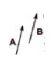### Negative Vectors:

Two vectors of equal magnitude but in opposite directions are called negative vectors.

###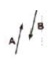Zero Vector or Null Vector:

A vector whose magnitude is zero is known as a zero or null Its direction is not defined. It is denoted by 0.
The velocity of a stationary object, acceleration of an object moving with uniform velocity and resultant of two equal and opposite vectors are the examples of the null vector.

### Unit Vector:

A vector having unit magnitude is called a unit vector. A unit vector in the direction of vector A is given by
Â = A / A
A unit vector is unit less and dimensionless vector and represents direction only.

### Position Vector:

A vector representing the straight line distance and the direction of any point or object with respect to the origin, is called position vector.

## Position and Displacement Vectors

To describe the position of an object moving in a plane, we need to choose a convenient point, say O as origin. Let P and P′ be the positions of the object at time t and t′ , respectively [Fig. 4.1(a)]. We join O and P by a straight line. Then, OP is the position vector of the object at time t. An arrow is marked at the head of this line. It is represented by a symbol r, i.e. OP = r. Point P′ is represented by another position vector, OP′ denoted by r′. The length of the vector r represents the magnitude of the vector and its direction is the direction in which P lies as seen from O. If the object moves from P to P′ , the vector PP′ (with tail at P and tip at P′ ) is called the displacement vector corresponding to motion from point P (at time t) to point P′ (at time t′ ) It is important to note that displacement
vector is the straight line joining the initial and final positions and does not depend on the actual path undertaken by the object between the two positions.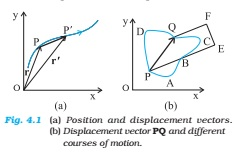Therefore, the magnitude of displacement is either less or equal to the path length of an object between two points.

We shall now describe this law of addition using the graphical method. Let us consider two vectors A and B that lie in a plane as shown in Fig. The lengths of the line segments representing these vectors are proportional to the magnitude of the vectors. To find the sum A + B, we place vector B so that its tail is at the head of the vector A, as in Fig. Then, we join the tail of A to the head of B. This line OQ represents a vector R, that is, the sum of the vectors A and B. Since, in this procedure of vector addition, vectors are arranged head to tail, this graphical method is called the head-to-tail method.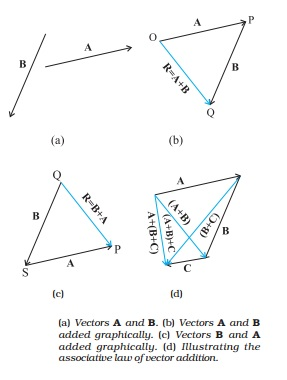### Triangle Law of Vectors:

If two vectors acting at a point are represented in magnitude and direction by the two sides of a triangle taken in one order, then their resultant is represented by the third side of the triangle taken in the opposite order.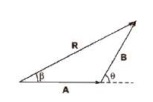If two vectors A and B acting at a point are inclined at an angle θ, then their resultant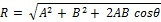If the resultant vector R subtends an angle β with vector A, then### Parallelogram Law of Vectors:

If two vectors acting at a point are represented in magnitude and direction by the two adjacent sides of a parallelogram draw from a point, then their resultant is represented in magnitude and direction by the diagonal of the parallelogram draw from the same point.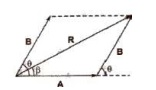Resultant of vectors A and B is given byIf the resultant vector R subtends an angle β with vector A, then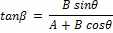i.e., A + B = B + A
i.e., A +(B + C)= B + (C + A)= C + (A + B)
i.e., m (A + B) = m A + mB

### Subtraction of Vectors:

Subtraction of a vector B from a vector A is defined as the addition of vector -B (negative of vector B) to vector A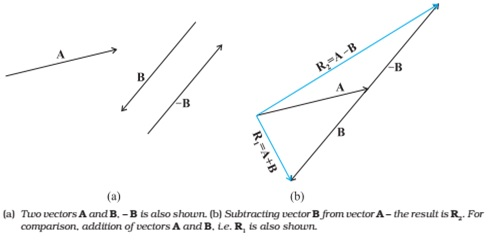Thus, A – B = A + (-B)

### Rotation of a Vector:

1. If a vector is rotated through an angle 0, which is not an integral multiple of 2 π, the vector
2. If the frame of reference is rotated or translated, the given vector does not change. The components of the vector may, however, change.

### Multiplication of a Vector by a Real Number:

When a vector A is multiplied by a real number n, then its magnitude becomes n times but direction and unit remain unchanged.